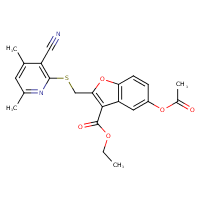Structure InformationCompound Identification

SMILES

CCOC(=O)C1=C(CSC2=C(C#N)C(C)=CC(C)=N2)OC2=C1C=C(OC(C)=O)C=C2

InChIKey

InChIKey=MAEBDSNJDPNJRA-UHFFFAOYSA-N

Formula

C22H20N2O5S

Mass

424.47